# VIPERS data exploration, visualization and analysis

Version 1.0, Bart Buelens, 25 January 2014.

www.astrostatistics.org

## Introduction

We explore galaxy redshift data collected through the VIPERS project. VIPERS stands for VIMOS Public Extragalactic Redshift Survey, and is an ESO Large Programme; VIMOS refers to Visible Imager Multi Object Spectrograph, an instrument on 'Melipal', the Very Large Telescope (VLT) Unit 3. In October 2013, the first Public Data Release (PDR-1) of the VIPERS project was released to the general public.

The author of this study has no involvement in the VIPERS project and is not affiliated with any of the VIPERS project partners. The analysis presented in this study is an example of the possibilities available today to hobby scientists in terms of working with data of professional quality collected through scientific observing campaigns.

The central point of entry for everything related to VIPERS is the VIPERS project website. This website provides access to a wealth of information, to the PDR-1 data sets, and to some relevant background papers.

More background information about the study presented here, and similar projects, can be found at www.astrostatistics.org. A working paper explaining the analyses presented here is forthcoming.

Acknowledgement This example uses data from the VIMOS Public Extragalactic Redshift Survey (VIPERS). VIPERS has been performed using the ESO Very Large Telescope, under the “Large Programme” 182.A-0886. The participating institutions and funding agencies are listed at http://vipers.inaf.it.

Initial operations needed to get started include loading some libraries we will need, and the data set. We use the spectroscopic data from the W4 field in this example.

library(ggplot2)
library(ks)  # kernel smoothing
library(deldir)  # Dirichlet (Voronoi) tessalation
datapath = "D:\\wherever your files are\\"
fileName = paste(datapath, "VIPERS_W4_SPECTRO_PDR1.txt", sep = "")
V = read.table(fileName, header = FALSE, dec = ".", row.names = NULL, comment.char = "#")
names(V) = c("VIPERS", "id_IAU", "num", "alpha", "delta", "selmag", "errselmag",
str(V)

'data.frame':   30698 obs. of  15 variables:
$VIPERS : Factor w/ 1 level "VIPERS": 1 1 1 1 1 1 1 1 1 1 ...$ id_IAU   : int  401019899 401031253 401013207 401026127 401027480 401011775 401023109 401024147 401039752 401021131 ...
$num : int 401019899 401031253 401013207 401026127 401027480 401011775 401023109 401024147 401039752 401021131 ...$ alpha    : num  330 330 330 330 330 ...
$delta : num 0.905 0.952 0.876 0.931 0.936 ...$ selmag   : num  22.2 20.8 21.6 21.4 21.5 ...
$errselmag: num 0.0391 0.0149 0.0219 0.0281 0.0424 0.0275 0.0263 0.0342 0.0392 0.0369 ...$ pointing : Factor w/ 93 levels "W4P001","W4P002",..: 1 1 1 1 1 1 1 1 1 1 ...
$quadrant : int 3 3 3 3 3 3 3 3 3 3 ...$ zflg     : num  2.5 3.5 0 2.5 0 1.5 2.5 1.5 3.5 2.5 ...
$zspec : num 0.819 0.819 10 0.724 10 ...$ epoch    : int  1 1 1 1 1 1 1 1 1 1 ...
$photoMask: int 1 1 1 1 1 1 1 1 1 1 ...$ tsr      : num  0.452 0.448 0.453 0.452 0.452 ...
$ssr : num 0.75 0.905 -1 0.903 -1 -1 0.864 -1 0.838 0.821 ...  We filter the data set to retain only those galaxies with reliable redshift measurements. Refer to the VIPERS project website and references from there for details about the variables occuring in the code below. dim(V)   30698 15  V = subset(V, ssr > 0 & tsr > 0) erf = function(x) { 2 * pnorm(x * sqrt(2)) - 1 } csrf = function(x) { b = 10.8 zt = 0.444 0.5 - 0.5 * erf(b * (zt - x)) } V$csr = csrf(V$zspec) V = subset(V, csr > 0) xtabs(~zflg, V)  zflg 2.2 2.4 2.5 3.2 3.4 3.5 4.2 4.4 4.5 9.2 9.4 9.5 1771 317 4067 1665 546 4517 1654 1119 5324 442 30 564  V = subset(V, zflg < 5 & zflg > 1) # retain only good quality data summary(V$zspec)

   Min. 1st Qu.  Median    Mean 3rd Qu.    Max.
0.0288  0.5770  0.6780  0.6960  0.7960  2.5800

qplot(V$zspec, binwidth = 0.03, colour = I("black"))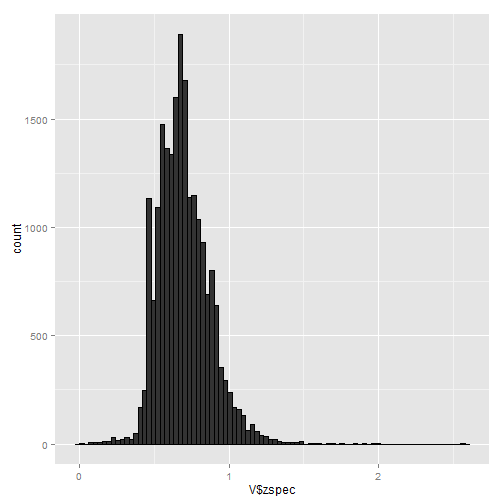# almost none at z > 1.2 V = subset(V, zspec >= 0.5 & zspec <= 1.2) dim(V)   18509 16   # calculate sampling weights: V$w = (1/V$ssr) * (1/V$tsr) * (1/V$csr) summary(V$w)

   Min. 1st Qu.  Median    Mean 3rd Qu.    Max.
2.06    2.51    2.69    2.77    2.95    4.76


## Data preprocessing

We project the data from right ascension, declination and redshift to a Cartesian system. While not strictly necessary, this will facilitate further analysis.

z2d = function(z) {
# crude linear approximation redshift to distance
c = 299792  # km/s
H = 100  # leave h =~ .71 within units ie. H = 100 h km /s / Mpc
d = z * c/H
return(d)  # unit Mpc/h
}

V$r = z2d(V$zspec)
summary(V$r)   Min. 1st Qu. Median Mean 3rd Qu. Max. 1500 1840 2100 2160 2430 3600  summary(V$alpha)  # in degrees, right ascension

   Min. 1st Qu.  Median    Mean 3rd Qu.    Max.
330     331     333     333     334     335

summary(V$delta) # in degrees, declination   Min. 1st Qu. Median Mean 3rd Qu. Max. 0.863 1.200 1.560 1.580 1.950 2.370   # distribution on celestial sphere: p = ggplot(V, aes(alpha, delta)) p + geom_point()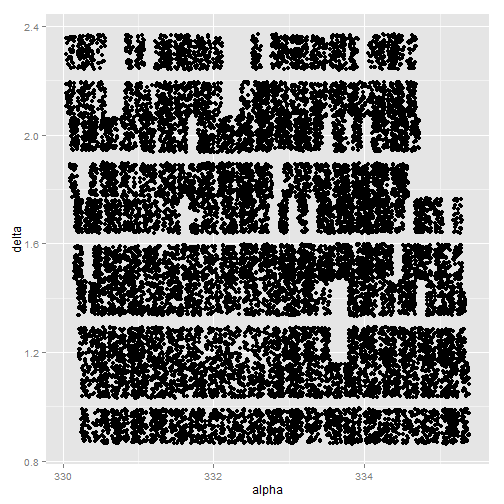# from degrees to radians: V$alpharad = (pi/180) * V$alpha V$deltarad = (pi/180) * V$delta # Cartesian coordinates: V$x = V$r * cos(V$deltarad) * cos(V$alpharad) V$y = V$r * cos(V$deltarad) * sin(V$alpharad) V$z = V$r * sin(V$deltarad)

# Project onto a plane through the observation volume, tilted to the mean
# declination of all galaxies, with the y-axis rotated to the mean right
# ascension
deltamean = mean(V$deltarad) alphamean = mean(V$alpharad)
V$xproj = V$r * cos(V$deltarad - deltamean) * cos(V$alpharad - alphamean)
V$yproj = V$r * cos(V$deltarad - deltamean) * sin(V$alpharad - alphamean)


## Visualization

Analysis of the galaxies will now proceed in the projection plane using the projected coordinates (xproj, yproj). We render the galaxies in this 2D plane in various ways. We select a rectangular window for convenience.


ggplot(subset(V, zspec > 0.6 & zspec < 0.9), aes(xproj, yproj)) + geom_point(size = 0.01)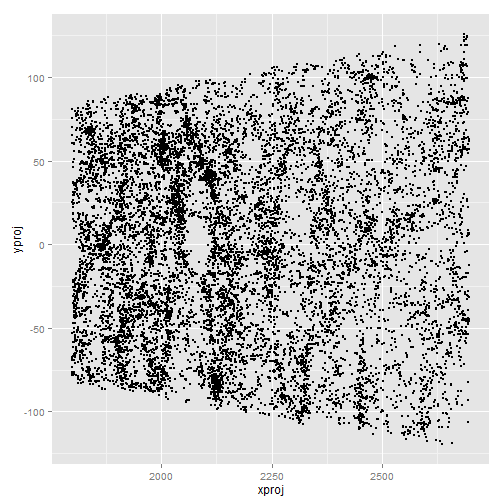# select a rectangular area for convenience:
xmin = 1850
xmax = 2300
ymin = -75
ymax = 75

VS = subset(V, xproj > xmin & xproj < xmax & yproj > ymin & yproj < ymax)
dim(V)

 18509    25

dim(VS)  # our selection

 6375   25

ggplot(VS, aes(xproj, yproj)) + geom_point(size = 0.01)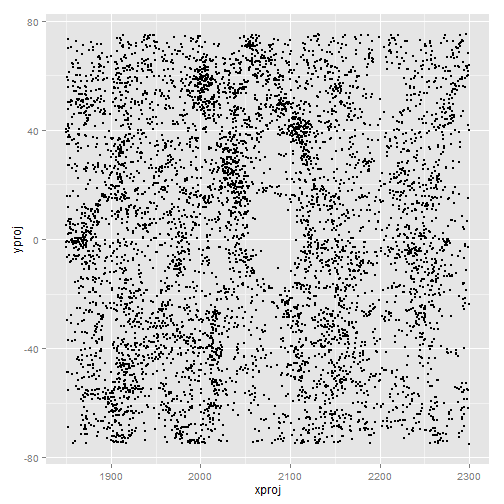# Visualization of VS in a nicer way
creme = "#FFFDD0"
mycolramp = colorRampPalette(c("black", creme))
mycol = densCols(VS[, c("xproj", "yproj")], colramp = mycolramp, nbin = 500)

oldpar = par()
par(bg = "black", col = "white", col.axis = "white", col.lab = "white", fg = "white")
smoothScatter(VS[, c("xproj", "yproj")], nrpoints = 0, colramp = mycolramp,
nbin = 500, transformation = function(x) x, bandwidth = 1.3)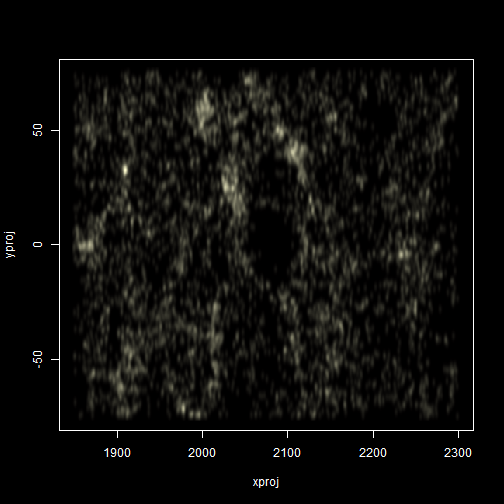par(oldpar)


## Spatial distribution analysis

Using kernel density estimation, two samples can be compared. The null hypothesis that both are genereated from the same density is tested. To be able to use this test, we generate a random realisation from the 2D uniform distribution. To verify that the test performs well, we test two independently generated unformly distributed samples.

# Is the distribution significantly different from uniform?
n = dim(VS)
Udf = data.frame(x = runif(n, min = min(VS$xproj), max = max(VS$xproj)), y = runif(n,
min = min(VS$yproj), max = max(VS$yproj)))
ggplot(Udf, aes(x, y)) + geom_point(size = 0.01)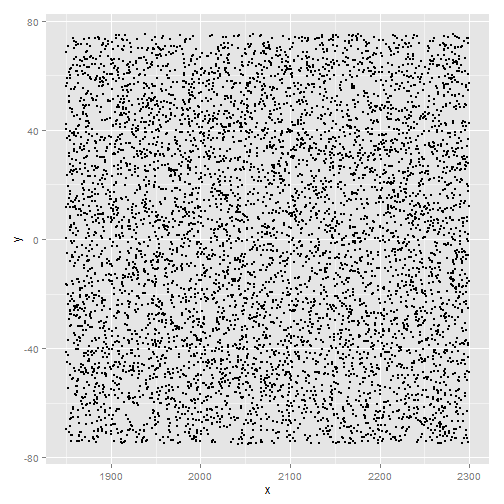X = kde.test(x1 = as.matrix(VS[, c("xproj", "yproj")]), x2 = as.matrix(Udf))
X$pvalue # highly significant!   4.443e-41   # double check this test, and test 2 uniformly distributed sets Udfbis = data.frame(x = runif(n, min = min(VS$xproj), max = max(VS$xproj)), y = runif(n, min = min(VS$yproj), max = max(VS$yproj))) Y = kde.test(x1 = as.matrix(Udf), x2 = as.matrix(Udfbis)) Y$pvalue  # not significant at all, good!

 0.7226


Going by this test and associated p-value, the null that both the uniformly distributed sample and the VIPERS sample follow the same distribution is rejected.

Further evidence that the spatial structure of the galaxies is not uniform is obtained in the following way. We obtain a Voronoi or Dirichlet partitioning, both for the VIPERS sample and for the uniform sample. We compare the distribution of the areas of the Voronoi cells.

# first partition our galaxies
VSlist = list(x = VS$xproj, y = VS$yproj)
VStess = deldir(VSlist, rw = c(xmin, xmax, ymin, ymax))

# plot a subset (plotting all is not nice, there are too many)
plot(VStess, xlim = c(2000, 2030), ylim = c(0, 30), wlines = "tess", pch = ".",
lty = "solid")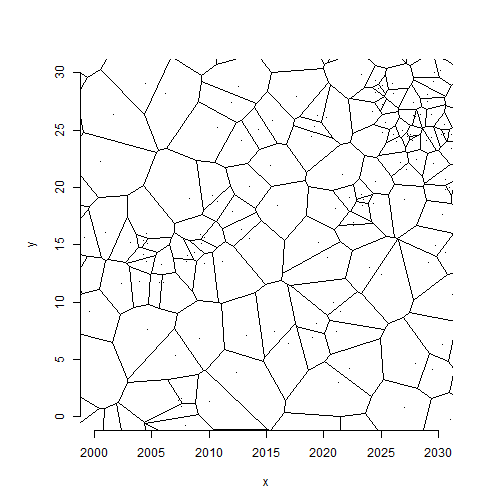# then process the uniform random sample
Ulist = list(x = Udf$x, y = Udf$y)
Utess = deldir(Ulist, rw = c(xmin, xmax, ymin, ymax))

# obtain areas of the dirichlet cells
VSa = VStess$summary$dir.area
Ua = Utess$summary$dir.area

summary(VSa)

   Min. 1st Qu.  Median    Mean 3rd Qu.    Max.
0.15    3.95    7.41   10.60   13.30  242.00

summary(Ua)

   Min. 1st Qu.  Median    Mean 3rd Qu.    Max.
0.41    6.45    9.57   10.60   13.70   46.60


n = length(VSa)
A = data.frame(source = c(rep("VIPERS", n), rep("Uniform", n)), area = c(VSa,
Ua))

qplot(A$area, fill = A$source, position = "dodge", xlim = c(0, 150), binwidth = 5)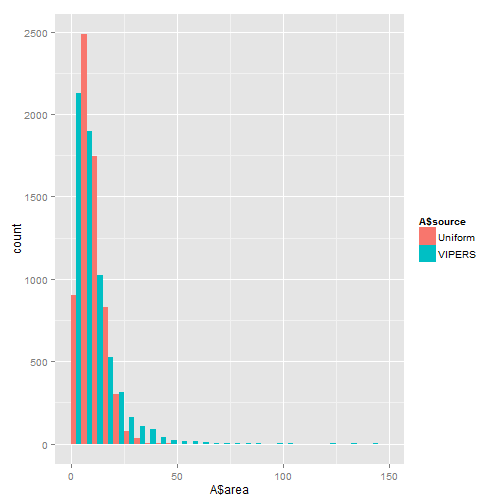qplot(A$area, fill = A$source, position = "dodge", xlim = c(0, 50), binwidth = 1)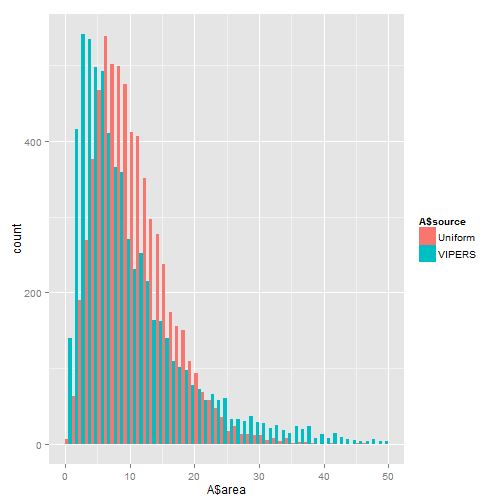ggplot(A, aes(area, fill = source)) + geom_density(alpha = 0.3) + xlim(0, 50)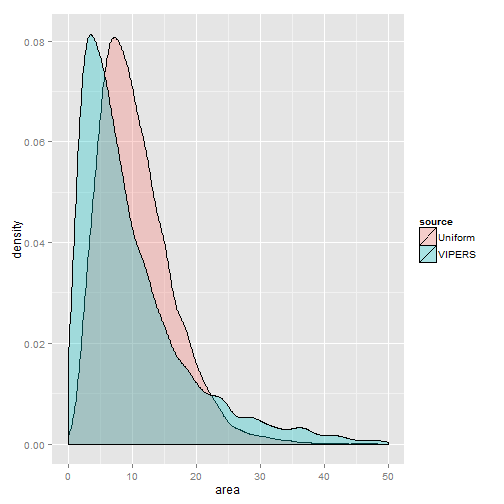These results will be further discussed and expanded upon in future. For more details and future updates please refer to www.astrostatistics.org.